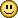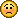cancel
Showing results for
Search instead for
Did you mean:

## AND having more trouble again

Champion
Posts: 4,158
Thanks: 626
Fixes: 5
Registered: ‎31-07-2007

## AND having more trouble again

May be in my seniority I'm losing my grip, so be kindIn what I want to do there are three possible conditions.
1.
A number in  cell A is less than cell X and greater than cell Y so in  cell B the result is contents cell A
2.
A number in  cell A is greater/equal than cell X so in  cell B the result is contents cell X
3.
A number in  cell A is smaller/equal than cell Y so in  cell B the result is contents cell Y
To deal with option 1 and use it as part of a nested IF,I tried "AND(A<X,A>Y)" expecting that both would return "TRUE", but only one did.
Where am I going wrong? It's embarrassing as I'm sure I have managed before.

No one has to agree with my opinion, but in the time I have left a miracle would be nice.
10 REPLIES 10
Grafter
Posts: 983
Registered: ‎06-06-2010

## Re: AND having more trouble again

if ( A < X AND A > Y)
B = A
else if( A >= X)
B = X
else if (A <= y)
B = y

is this right ?
Grafter
Posts: 983
Registered: ‎06-06-2010

## Re: AND having more trouble again

replacing

AND
with its token in whatever pc language your using
in ansi c  AND is
||
Grafter
Posts: 523
Thanks: 2
Registered: ‎17-07-2008

## Re: AND having more trouble again

 Quote from: giro in ansi c  AND is     ||
I think you meant to write && (|| is logical OR)Grafter
Posts: 983
Registered: ‎06-06-2010

## Re: AND having more trouble again

oops yes MrC is  correct
AND in c is &&
sorry
Champion
Posts: 4,158
Thanks: 626
Fixes: 5
Registered: ‎31-07-2007

## Re: AND having more trouble again

Is much of what pps have written e.g, elseif VBA, as I don't have such a function in my 2007 program?
VBA is  terra incognita for me,, so plain Excel pse.No one has to agree with my opinion, but in the time I have left a miracle would be nice.
Grafter
Posts: 983
Registered: ‎06-06-2010

## Re: AND having more trouble again

IF condition1 THEN
value_if_true1
ELSEIF condition2 THEN
value_if_true2
ELSE
value_if_false2
END IF
so example
In Excel, you need to write a formula that works this way:
If (cell A1) is less than 20, then multiply by 1,
If it is greater than or equal to 20 but less than 50, then multiply by 2
If its is greater than or equal to 50 and less than 100, then multiply by 3
And if it is great or equal to than 100, then multiply by 4
Answer:  You can write a nested IF statement to handle this. For example:
=IF(A1<20, A1*1, IF(A1<50, A1*2, IF(A1<100, A1*3, A1*4)))
Grafter
Posts: 983
Registered: ‎06-06-2010

## Re: AND having more trouble again

another example using AND
Question: In Excel, I need a formula in cell C5 that does the following:
IF A1+B1 <= 4, return 20
IF A1+B1 > 4 but <= 9, return 35
IF A1+B1 > 9 but <= 14, return 50
IF A1+B1 > 15, return 75
you can write a nested IF statement that uses the AND function as follows:
=IF((A1+B1)<=4,20,IF(AND((A1+B1)>4,(A1+B1)<=9),35,IF(AND((A1+B1)>9,(A1+B1)<=14),50,75)))
Grafter
Posts: 983
Registered: ‎06-06-2010

## Re: AND having more trouble again

but

IF condition1 THEN
value_if_true1
ELSEIF condition2 THEN
value_if_true2
ELSE
value_if_false2
END IF
This syntax example demonstrates how to nest two IF functions. You can nest up to 7 IF functions.
condition is the value that you want to test.
value_if_true is the value that is returned if condition evaluates to TRUE.
value_if_false is the value that is return if condition evaluates to FALSE.
Applies To:
* Excel 2007, Excel 2003, Excel XP, Excel 2000
so you do have the easier method in excel
Community Veteran
Posts: 38,460
Thanks: 1,032
Fixes: 62
Registered: ‎15-06-2007

## Re: AND having more trouble again

=IF(AND(A1<B1,A1>C1),A1,IF(OR(A1>B1,A1=B1),B1,C1))
Where A1 = cell A
Where B1 = cell X
Where C1 = cell Y
Grafter
Posts: 983
Registered: ‎06-06-2010

THANKS JIM